# PARALLEL AND PERPENDICULAR LINES Slopes Parallel Lines The

• Slides: 22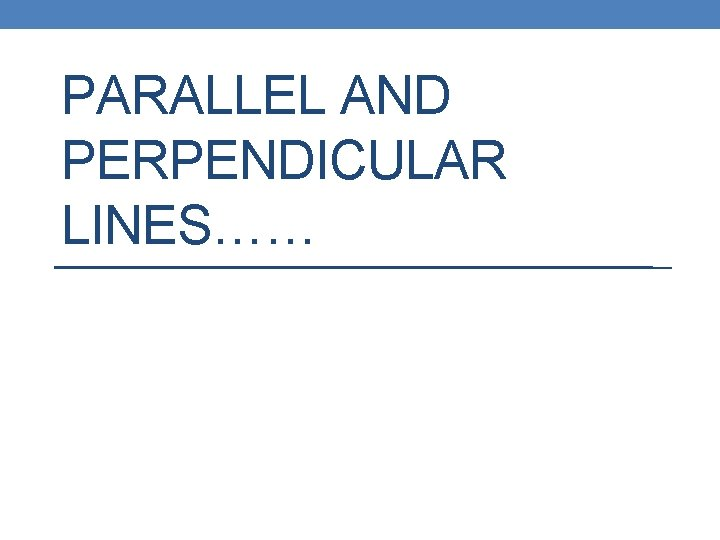PARALLEL AND PERPENDICULAR LINES……Slopes…… • Parallel Lines: The slopes are EQUAL for parallel lines. • Perpendicular Lines: The slopes are opposite reciprocals for perpendicular lines.Example……If • PARALLEL: • PERPENDICULAR: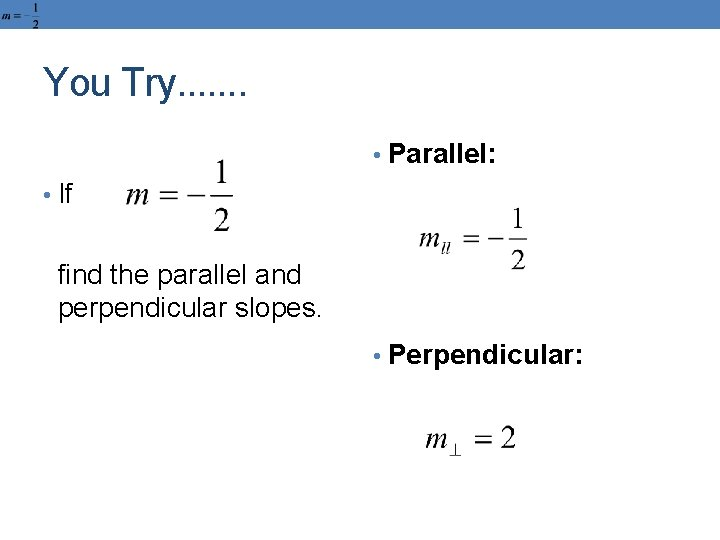You Try. . . . • Parallel: • If find the parallel and perpendicular slopes. • Perpendicular: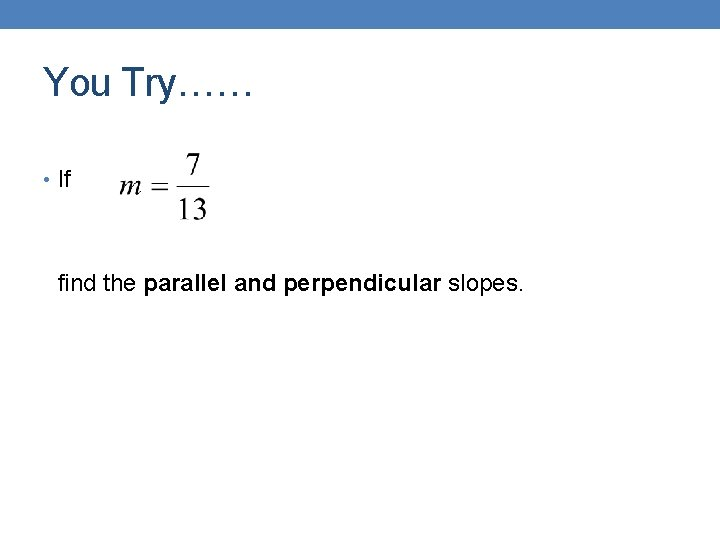You Try…… • If find the parallel and perpendicular slopes.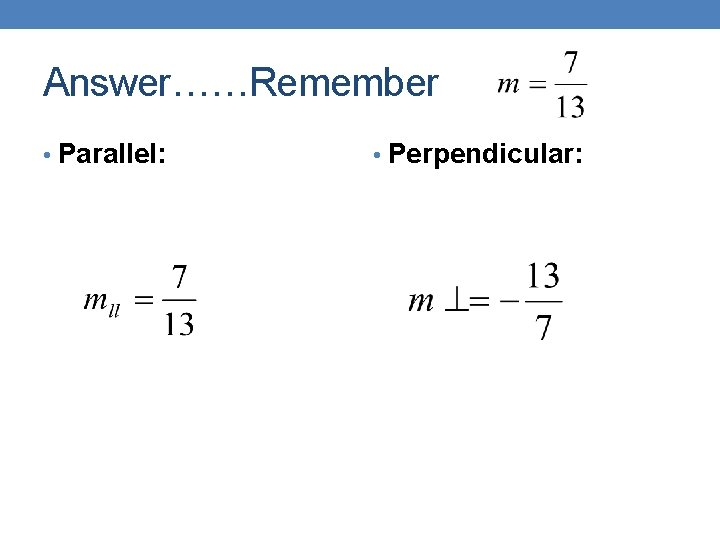Answer……Remember • Parallel: • Perpendicular:Example…… • A. What is the slope of • A. ? • B. What is the parallel • B. • C. What is the • C. slope? perpendicular slope?Example…… • Graph the line parallel to through (8, 2).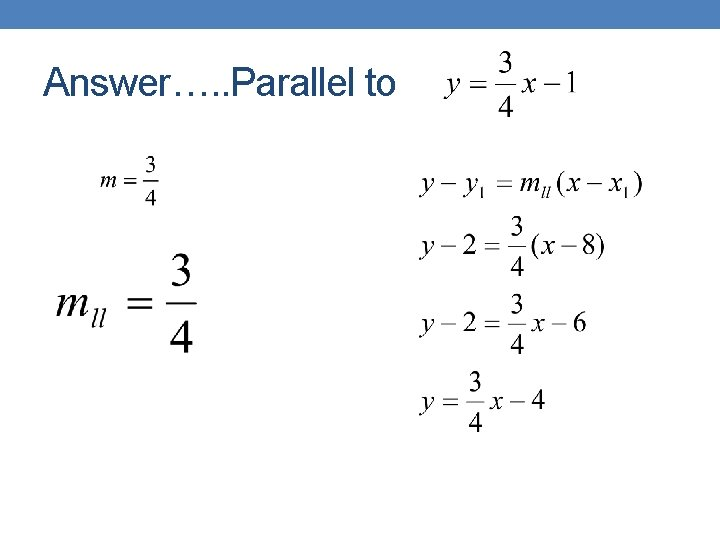Answer…. . Parallel to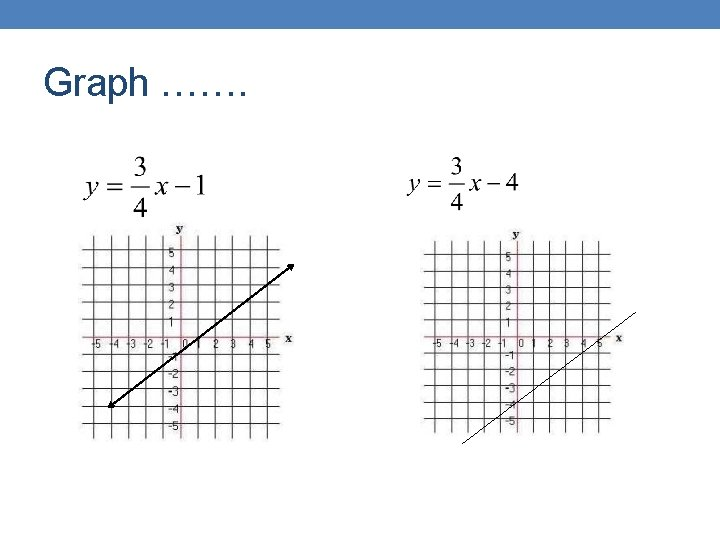Graph …….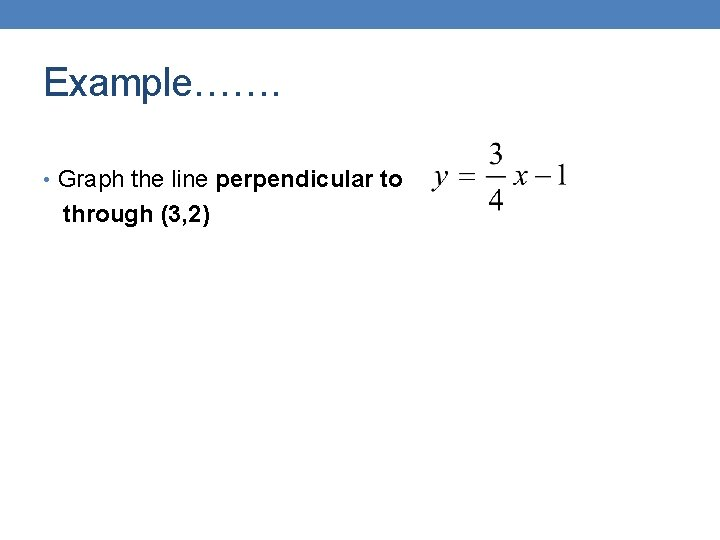Example……. • Graph the line perpendicular to through (3, 2)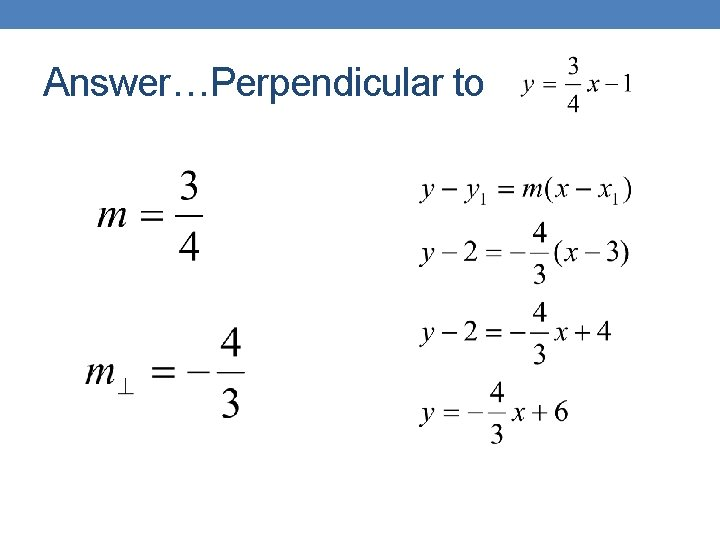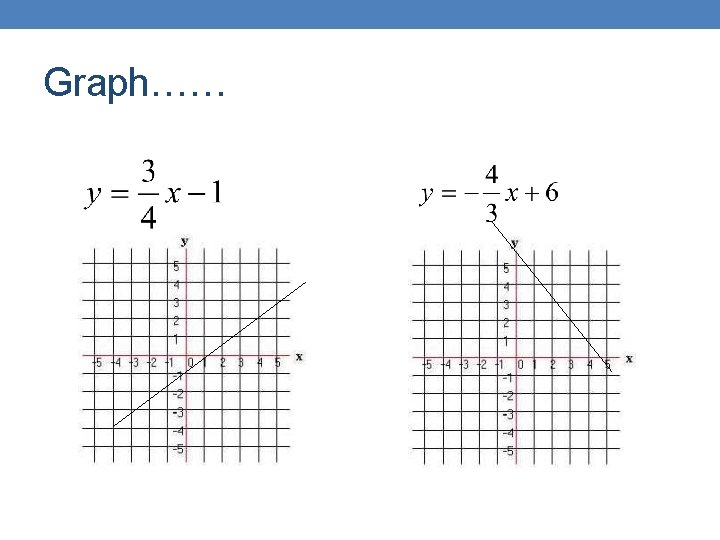Graph……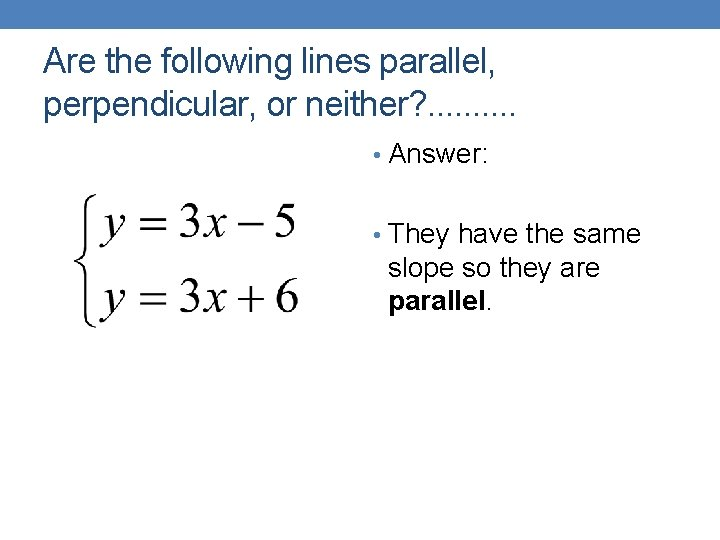Are the following lines parallel, perpendicular, or neither? . . • Answer: • They have the same slope so they are parallel.Parallel, Perpendicular, or Neither? • The slopes are not the same so they are NOT parallel. • The slopes are not opposite reciprocals so they are NOT perpendicular. • They are neither.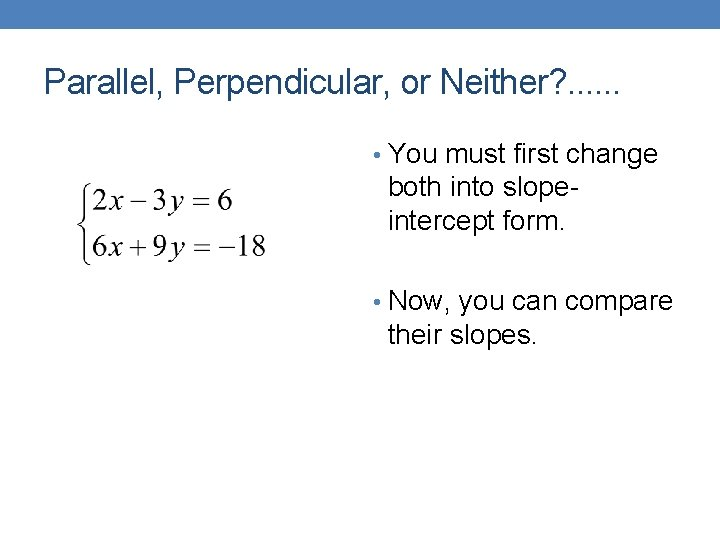Parallel, Perpendicular, or Neither? . . . • You must first change both into slopeintercept form. • Now, you can compare their slopes.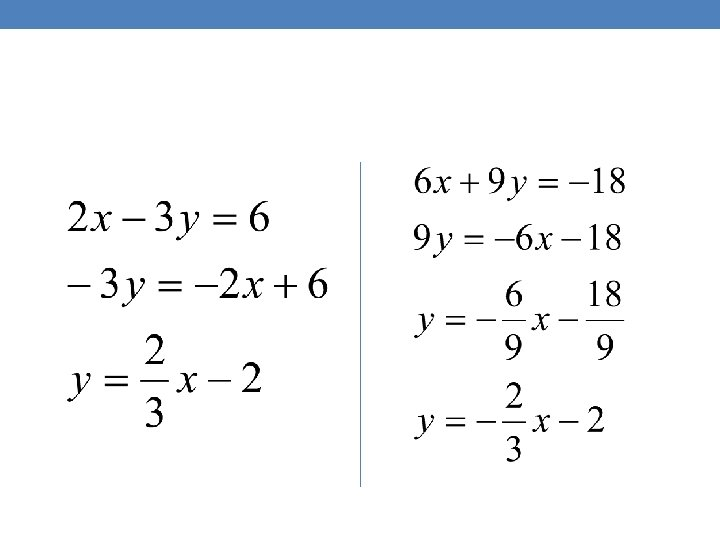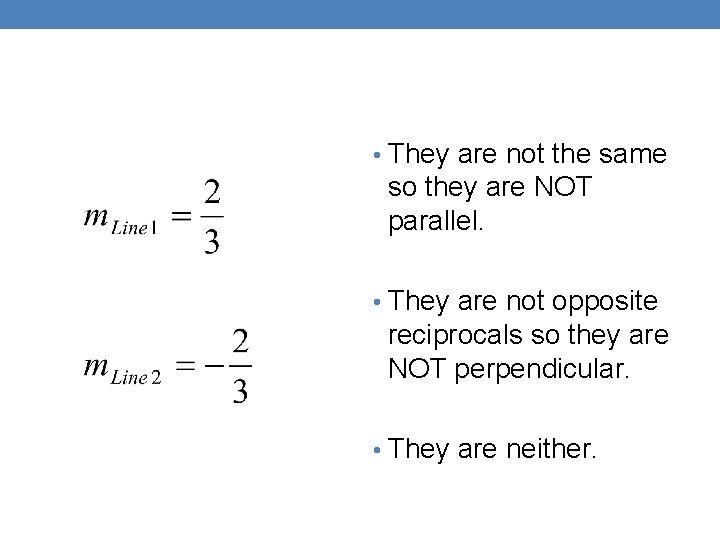• They are not the same so they are NOT parallel. • They are not opposite reciprocals so they are NOT perpendicular. • They are neither.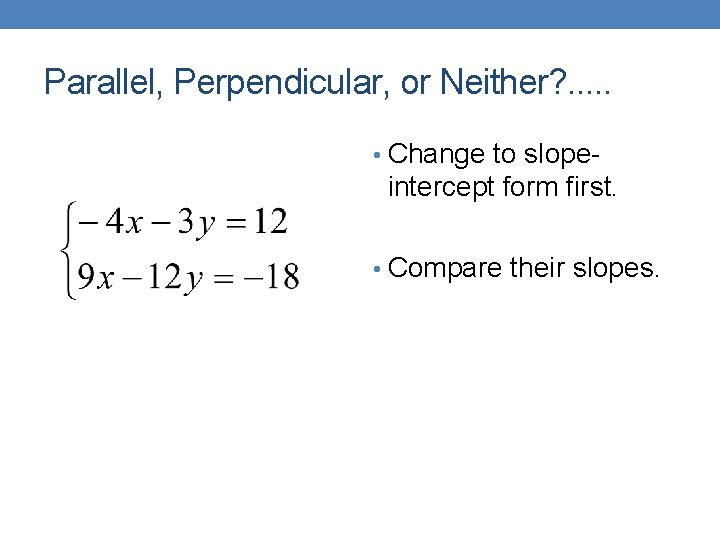Parallel, Perpendicular, or Neither? . . . • Change to slope- intercept form first. • Compare their slopes.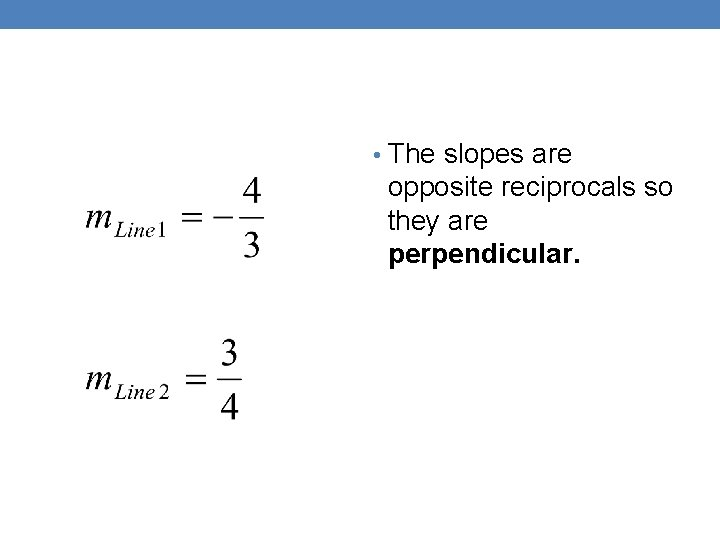• The slopes are opposite reciprocals so they are perpendicular.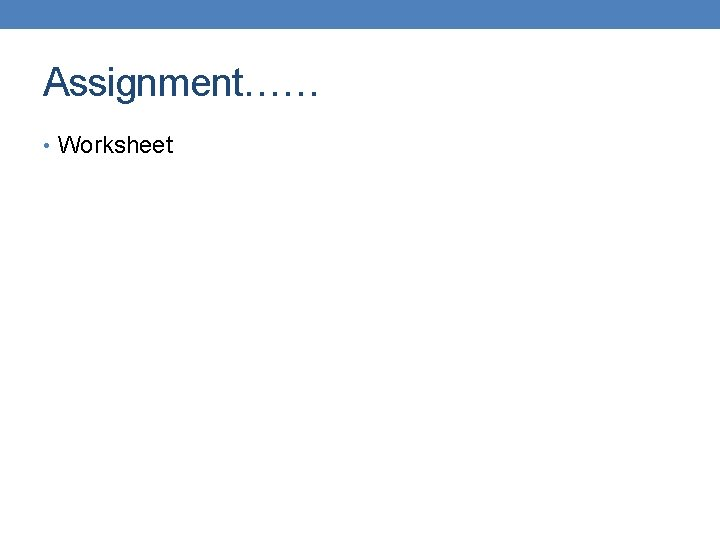Assignment…… • Worksheet